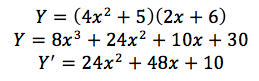### Differentiation Rules

#### Product Rule

###### Where is it used?

The product rule is used where an equation that requires differentiation consists of two separate equations multiplied together. It is a quicker way to solve a problem then to simply expand and then differentiate.

###### What is the Formula?Where g(x) is equation 1 and f(x) is equation 2 and g'(x) is the differentiation of g(x) and f'(x) is the differentiation of f(x).

Worked Example

In this example, we will try to differentiate Y=(4x^2+5)(2x+6)

Step 1: Determine which equation will g(x) and f(x) and then determine g'(x) and f'(x). Any equation can be g(x) or f(x). This is shown for demonstration purposes. When completing an assessment you do not need to show this step to receive full marks.Step 2: Substitute g(x), g'(x), f(x) and f'(x) into the product rule formula.Worked Example But Through Expansion

To show that our answer we received is correct, we will differentiate by expanding the equation first.Unless specifically stated, you will still get full marks for differentiation through expansion first. However, it is likely you will get equations where expansion would be difficult without a calculator, hence, product rule would be a preferred option.